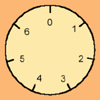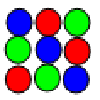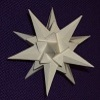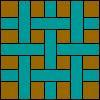# Resources tagged with: Modular arithmetic

Filter by: Content type:
Age range:
Challenge level:

### There are 39 results

Broad Topics > Numbers and the Number System > Modular arithmetic### Old Nuts

##### Age 16 to 18 Challenge Level:

In turn 4 people throw away three nuts from a pile and hide a quarter of the remainder finally leaving a multiple of 4 nuts. How many nuts were at the start?### Modulus Arithmetic and a Solution to Dirisibly Yours

##### Age 16 to 18

Peter Zimmerman from Mill Hill County High School in Barnet, London gives a neat proof that: 5^(2n+1) + 11^(2n+1) + 17^(2n+1) is divisible by 33 for every non negative integer n.### More Sums of Squares

##### Age 16 to 18

Tom writes about expressing numbers as the sums of three squares.### Dirisibly Yours

##### Age 16 to 18 Challenge Level:

Find and explain a short and neat proof that 5^(2n+1) + 11^(2n+1) + 17^(2n+1) is divisible by 33 for every non negative integer n.### The Public Key

##### Age 16 to 18 Challenge Level:

Find 180 to the power 59 (mod 391) to crack the code. To find the secret number with a calculator we work with small numbers like 59 and 391 but very big numbers are used in the real world for this.### Remainder Hunt

##### Age 16 to 18 Challenge Level:

What are the possible remainders when the 100-th power of an integer is divided by 125?### Elevens

##### Age 16 to 18 Challenge Level:

Add powers of 3 and powers of 7 and get multiples of 11.### Modulus Arithmetic and a Solution to Differences

##### Age 16 to 18

Peter Zimmerman, a Year 13 student at Mill Hill County High School in Barnet, London wrote this account of modulus arithmetic.### Novemberish

##### Age 14 to 16 Challenge Level:

a) A four digit number (in base 10) aabb is a perfect square. Discuss ways of systematically finding this number. (b) Prove that 11^{10}-1 is divisible by 100.### Prime AP

##### Age 16 to 18 Challenge Level:

What can you say about the common difference of an AP where every term is prime?### The Chinese Remainder Theorem

##### Age 14 to 18

In this article we shall consider how to solve problems such as "Find all integers that leave a remainder of 1 when divided by 2, 3, and 5."### Sixinit

##### Age 16 to 18 Short Challenge Level:

Choose any whole number n, cube it, add 11n, and divide by 6. What do you notice?### An Introduction to Modular Arithmetic

##### Age 14 to 16

An introduction to the notation and uses of modular arithmetic### Double Time

##### Age 16 to 18 Challenge Level:

Crack this code which depends on taking pairs of letters and using two simultaneous relations and modulus arithmetic to encode the message.### Latin Squares

##### Age 11 to 18

A Latin square of order n is an array of n symbols in which each symbol occurs exactly once in each row and exactly once in each column.### Modular Fractions

##### Age 16 to 18 Challenge Level:

We only need 7 numbers for modulus (or clock) arithmetic mod 7 including working with fractions. Explore how to divide numbers and write fractions in modulus arithemtic.### Obviously?

##### Age 14 to 18 Challenge Level:

Find the values of n for which 1^n + 8^n - 3^n - 6^n is divisible by 6.### Pythagoras Mod 5

##### Age 16 to 18 Challenge Level:

Prove that for every right angled triangle which has sides with integer lengths: (1) the area of the triangle is even and (2) the length of one of the sides is divisible by 5.### Small Groups

##### Age 16 to 18

Learn about the rules for a group and the different groups of 4 elements by doing some simple puzzles.##### Age 16 to 18 Challenge Level:

Decipher a simple code based on the rule C=7P+17 (mod 26) where C is the code for the letter P from the alphabet. Rearrange the formula and use the inverse to decipher automatically.### The Knapsack Problem and Public Key Cryptography

##### Age 16 to 18

An example of a simple Public Key code, called the Knapsack Code is described in this article, alongside some information on its origins. A knowledge of modular arithmetic is useful.### Mod 7

##### Age 16 to 18 Challenge Level:

Find the remainder when 3^{2001} is divided by 7.### Happy Birthday

##### Age 16 to 18 Challenge Level:

Can you interpret this algorithm to determine the day on which you were born?### Filling the Gaps

##### Age 14 to 16 Challenge Level:

Which numbers can we write as a sum of square numbers?### More Mods

##### Age 14 to 16 Challenge Level:

What is the units digit for the number 123^(456) ?### Mod 3

##### Age 14 to 16 Challenge Level:

Prove that if a^2+b^2 is a multiple of 3 then both a and b are multiples of 3.### Zeller's Birthday

##### Age 14 to 16 Challenge Level:

What day of the week were you born on? Do you know? Here's a way to find out.### Take Three from Five

##### Age 14 to 16 Challenge Level:

Caroline and James pick sets of five numbers. Charlie chooses three of them that add together to make a multiple of three. Can they stop him?### Purr-fection

##### Age 16 to 18 Challenge Level:

What is the smallest perfect square that ends with the four digits 9009?### Check Codes

##### Age 14 to 16 Challenge Level:

Details are given of how check codes are constructed (using modulus arithmetic for passports, bank accounts, credit cards, ISBN book numbers, and so on. A list of codes is given and you have to check. . . .### Grid Lockout

##### Age 14 to 16 Challenge Level:

What remainders do you get when square numbers are divided by 4?### Knapsack

##### Age 14 to 16 Challenge Level:

You have worked out a secret code with a friend. Every letter in the alphabet can be represented by a binary value.### Guesswork

##### Age 14 to 16 Challenge Level:

Ask a friend to choose a number between 1 and 63. By identifying which of the six cards contains the number they are thinking of it is easy to tell them what the number is.### Check Code Sensitivity

##### Age 14 to 16 Challenge Level:

You are given the method used for assigning certain check codes and you have to find out if an error in a single digit can be identified.### Odd Stones

##### Age 14 to 16 Challenge Level:

On a "move" a stone is removed from two of the circles and placed in the third circle. Here are five of the ways that 27 stones could be distributed.### Rational Round

##### Age 16 to 18 Challenge Level:

Show that there are infinitely many rational points on the unit circle and no rational points on the circle x^2+y^2=3.### Transposition Fix

##### Age 14 to 16 Challenge Level:

Suppose an operator types a US Bank check code into a machine and transposes two adjacent digits will the machine pick up every error of this type? Does the same apply to ISBN numbers; will a machine. . . .### Euler's Officers

##### Age 14 to 16 Challenge Level:

How many different ways can you arrange the officers in a square?### The Best Card Trick?

##### Age 11 to 16 Challenge Level:

Time for a little mathemagic! Choose any five cards from a pack and show four of them to your partner. How can they work out the fifth?